# 防御力

## 机制

### 普通模式公式

`净伤害 = 攻击伤害 - (防御力 * 0.5)`

• 如果有 20 防御力，一次 40 伤害的攻击会被降低 10，结果是承受 30 伤害：`40 - (20 * 0.5) = 30`
• 如果有 30 防御力，一次 40 伤害的攻击会被降低 15，结果是承受 25 伤害：`40 - (30 * 0.5) = 25`

### 专家模式公式

`净伤害 = 攻击伤害 - (防御力 * 0.75)`

• 如果有 20 防御力，一次 40 伤害的攻击会被降低 15，结果是承受 25 伤害：`40 - (20 * 0.75) = 25`
• 如果有 30 防御力，一次 40 伤害的攻击会被降低 23，结果是承受 17 伤害：`40 - (30 * 0.75) = 17`

## 敌怪

`净伤害 = 攻击伤害 - (防御力 * 0.5)`

## 改变防御力

### 盔甲和配饰

1
1
1克苏鲁护盾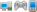2
2
3（仅在夜晚期间）
3（仅限夜晚期间）
4（仅限夜晚期间）
4（仅限白天期间）
4
4
6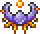天界壳4（白天期间）
7（夜晚期间）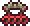血肉指关节7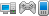0，直到低于 50% 生命值，此时会降低 25% 总伤害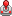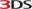30（当低于 25% 生命值）

### 增益和减益

2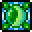树妖祝福8
8
-4
-4
-20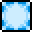双足翼龙诅咒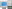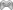-40
-50%

## 伤害减免强化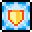太阳烈焰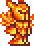耀斑盔甲30%
15% / 30% / 45%
25%（仅当生命值高于 25% 时有效）
10%25%（当低于 50% 生命值时）0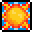温暖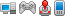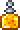保暖药水30%（只会减少来冷系敌怪的伤害）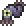蠕虫围巾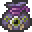宝藏袋（世界吞噬怪）17%

## 盔甲穿透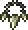鲨牙项链5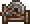利器站4（仅对近战武器

## 备注

### 防御力

#### 电脑版和主机版

```79 + 28 + 18 + 20 + 7 = 152 (普通模式)
79 + 48 + 18 + 20 + 7 = 172 (普通模式加上{{tr|Warding modifiers}})
79 + 32 + 18 + 20 + 7 = 156 (专家模式)
79 + 56 + 18 + 20 + 7 = 180 (专家且加上{{tr|Warding armor}}组件)
```

#### 移动版

```79 + 28 + 18 + 20 + 7 = 152 (普通模式)
79 + 48 + 18 + 20 + 7 = 172 (普通模式加上{{tr|Warding modifiers}})
79 + 32 + 18 + 20 + 7 = 156 (专家模式)
79 + 56 + 18 + 20 + 7 = 180 (专家且加上{{tr|Warding armor}}组件)
```

#### Old Gen Console和3DS版

```76 + 22 + 10 + 20 = 128 (普通模式)
76 + 42 + 10 + 20 = 148 (普通模式加上{{tr|Warding modifiers}})
```

### 伤害减免

#### 电脑版、主机版、和移动版

```(1 - 0.45) * (1 - (0.25 + 0.17 + 0.1) ) = 0.264 = 承受 26.4% 伤害 = 减免 73.6% 伤害

(1 - 0.45) * (1 - (0.25 + 0.17 + 0.1 + 0.3) ) = 0.099 = 承受 9.9% 伤害 = 针对冷系{{tr|enemy}}减免 91.1% 伤害```

#### Old Gen Console Version

• 盔甲（乘算）
• 配饰和药水（加算）
• 耐力药水 – 10% 减免
• 保暖药水 - 针对 冷系敌怪 30% 减免
• 这不包括圣骑士护盾增益，因为其伤害减免是发生在计算防御之前，而其他都是在计算防御之后发生的。
```(1 - 0.45) * (1 - 0.1) = 0.495 = 承受 49.5% 伤害 = 减免 50.5% 伤害

(1 - 0.45) * (1 - (0.1 + 0.3) ) = 0.33 = 承受 33% 伤害 = 针对冷系{{tr|enemy}}减免 66% 伤害```

#### 3DS版

• 盔甲（乘算）
• 配饰和药水（加算）
• 耐力药水 – 10% 减免
• 这不包括圣骑士护盾增益，因为其伤害减免是发生在计算防御之前，而其他都是在计算防御之后发生的。
`(1 - 0.45) * (1 - 0.1) = 0.495 = 承受 49.5% 伤害 = 减免 50.5% 伤害`

## 花絮

• 虽然在游戏中不可能，但如果每件盔甲都能获得一个修饰语，它就会像配饰那样应用效果。例如，一整套有护佑修饰语的盔甲将总共增加 12 防御；每个单件都会为总量贡献 4 防御。
• 虽然专家模式下的伤害减免是总防御力的四分之三，但专家模式下的敌怪伤害却翻倍了，这使得专家模式下的伤害减免效果依然不及普通模式。
• 如果玩家以某种方式具有负防御，他们将持续受到 1 点伤害，并且在死亡时会有死亡讯息“<playername>无法把火扑灭。"